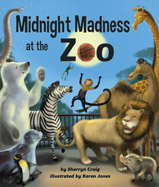Alignment to Standards for TX1 111.13 (1.1) uses whole numbers to describe and compare quantities.
1 111.13 (1.3) recognizes and solves problems in addition and subtraction situations.
2 111.14 (2.3) (A) is expected to recall and apply basic addition and subtraction facts to 18.
K 111.12 (K.1) uses numbers to name quantities.
K 111.12 (K.1) (A) is expected to use one-to-one correspondence and language such as more than, same number as, or two less than to describe relative sizes of sets of concrete objects.
K 111.12 (K.1) (C) is expected to use numbers to describe how many objects are in a set (through 20) using verbal and symbolic descriptions.
PK PK.1. (B) counts by ones to 10 or higher
PK PK.1. (C) counts concrete objects to five or higher
PK PK.1. (D) begins to compare the numbers of concrete objects using language (e.g., ''same'' or ''equal,'' ''one more,'' ''more than,'' or ''less than'')
PK PK.1. (E) begins to name ''how many'' are in a group of up to three (or more) objects without counting (e.g., recognizing two or three crayons in a box)

Back to Standards Page